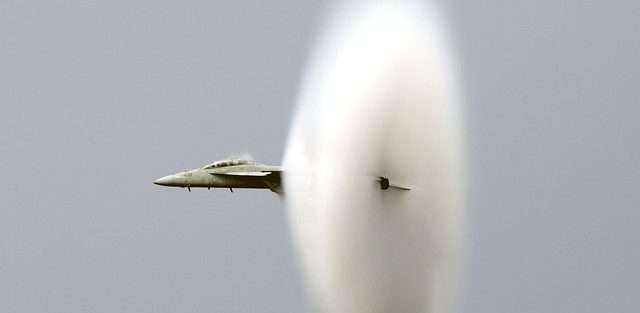# Speed of SoundThe speed of sound is the speed at which sound travels, which is equivalent to 761 mph at sea level. The speed of sound also affects aircraft forces, and the relation between the two is called Mach speed. By definition, however, Mach 1 is equal to the speed of sound.

## Mach Speed in Aerodynamics

Mach number, which is a useful quantity when it comes to aerodynamics, is determined by the ratio of speed of sound to air speed. There are three terms to help describe the speed of an airborne aircraft:

• Subsonic – an aircraft moving slower than the speed of sound
• Trans sonic – an aircraft moving close to or slightly faster than the speed of sound
• Supersonic – an aircraft moving faster than the speed of sound

## Calculating the Mach Number

Because the mach number is a ratio of speed of sound to air speed, the mach number is determined by dividing the speed of the aircraft by the speed of sound in the medium.

For example, Mach 0.65 is 65 percent of the speed of sound, Mach 1 is equal to the speed of sound, and Mach 1.35 is 35 percent faster than the speed of sound.

There are a few factors to consider when looking for the Mach number. The medium that surrounds the speed of sound can alter the results, in particular the temperature.